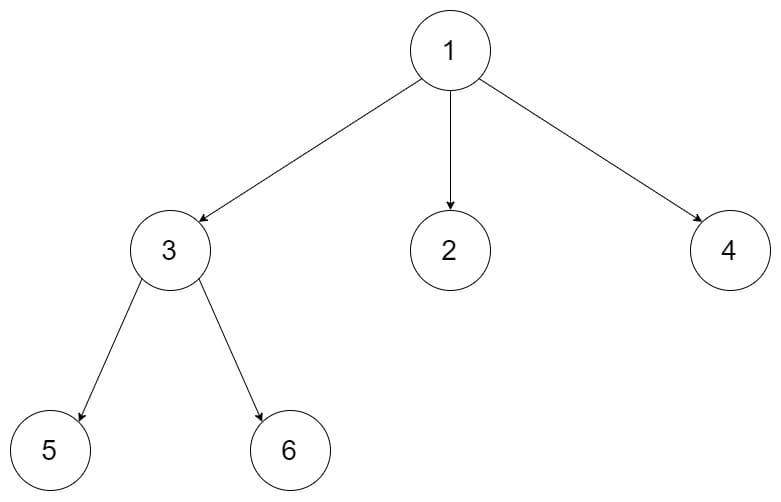# 589. N-ary Tree Preorder Traversal

Reference: LeetCode
Difficulty: Easy

## Problem

Given an n-ary tree, return the preorder traversal of its nodes’ values.

Example:Follow up: Recursive solution si trivial, could you do it iteratively?

## Analysis

### Recursion

Time: $O(N)$
Space: $O(h)$

### Iteration

Note:

• Notice that if the children list is implemented with LinkedList, we’d better use Collections.reverse() to reverse it rather than using get, which might take $O(N^2)$ runtime in the worst case.
• Also notice that children list is guaranteed to be not null, and no element is null too.

Time: $O(N)$
Space: $O(N)$. As for N-ary trees, the iteration method’s runtime is determined by the maximum number of nodes at any level. See the worst case example below (also consider the result list).

The worst case of space is as follows:

First level contains only the root, while all other nodes lie in the second level. So we need to push all the nodes in the list.

CommentJunhao Wang
a software engineering cat